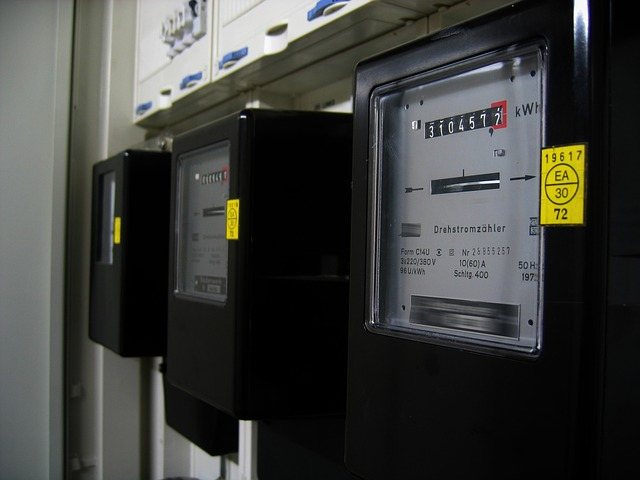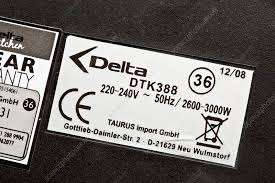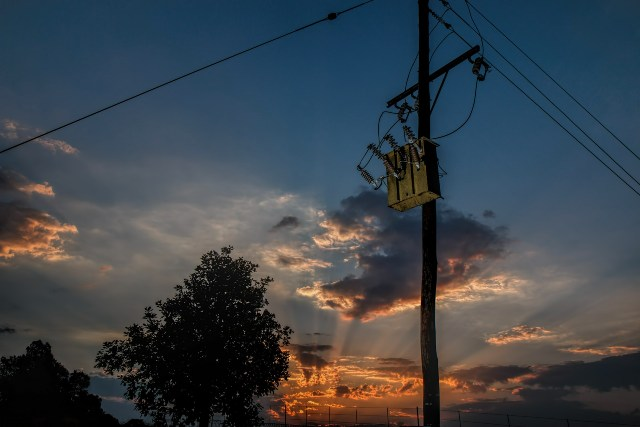Select Page

# The Ultimate Guide

written by Stanley Udegbunam || Dec 30, 2020#### AFRILCATE

Electricity is measured in different units of power which include the watt, kilowatt and megawatt.

## Watts

This is the smallest unit measurement of electric power.

Household electrical appliances like electric iron and water heater have electrical rating plates on them.

The plate outlines the power rating of the appliance in Watts.electricity rating plate or power nameplate

## Kilowatt (KW)

Due to high power demand from electrical appliances, the power rating is expressed in kilowatt (KW) rather than Watt(W).

 1KW= 1000W

Hence for the electric kettle above, 3,000W = 3KW

Some home electric showers consume up to 10.5 KW

## Megawatt (MW)

It is a much larger amount of energy used to measure the power output of cities, power plants and large factories.

 1MW= 1000KW  = ( 1 million watt)

Palo Verde nuclear power plant in Arizona is the largest nuclear power plant in the United States.

The power plant is endowed with three reactors and total net summer electricity generating capacity of about 3,937 MW.

Tokyo Electric Power Co.’s (TEPCO) Kashiwazaki-Kariwa plant in Japan is currently the world’s largest nuclear power plant.

It has a net capacity of 7,965MW.

## Gigawatt (GW)

Gigawatt is suitable for measuring the capacity of large power plants or power grid.

 1GW= 1000MW

100 MW = 1 million Kilowatt = 1 billion watt

therefore, 1 GW = 1 billion watt

 1GW= 1000MW

100 MW = 1 million Kilowatt = 1 billion watt

therefore, 1 GW = 1 billion watt

In 2019, U.S nuclear generation capacity exceeded more than 98 gigawatts.AFRILCATE article – how is electricity measured?

## ELECTRICITY MEASUREMENT SUMMARY

Electricity production and consumption are most commonly measured in kilowatt-hours (kWh).

A kilowatt-hour means one kilowatt (1,000 watts) of electricity produced or consumed for one hour.

It indicates the amount of energy that would be expended if a 1,000-watt appliance runs for an hour:

 1KWH= 1000W in 1 HR

So if you switched on a 50 watt light bulb, it would take 20 hours to expend 1 kWh of energy.

A  2,500-watt electric kettle would use 1 kWh in just 25 minutes.

Whereas a 4000-watt cooker utilized for 1 hour will consume 4 kWh of electricity.

Electricity bill usually indicates the number of kilowatt-hours (kWh) consumed by households during the period stated on the bill.

In 2018, the average annual electricity consumption for a U.S. residential utility customer was 10,972 kilowatt-hours (kWh).

This amounts to an average of about 914 kWh per month.